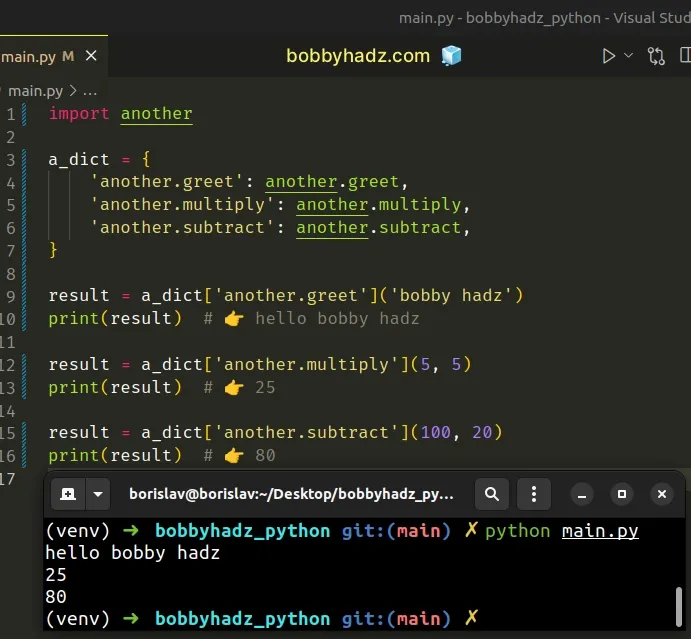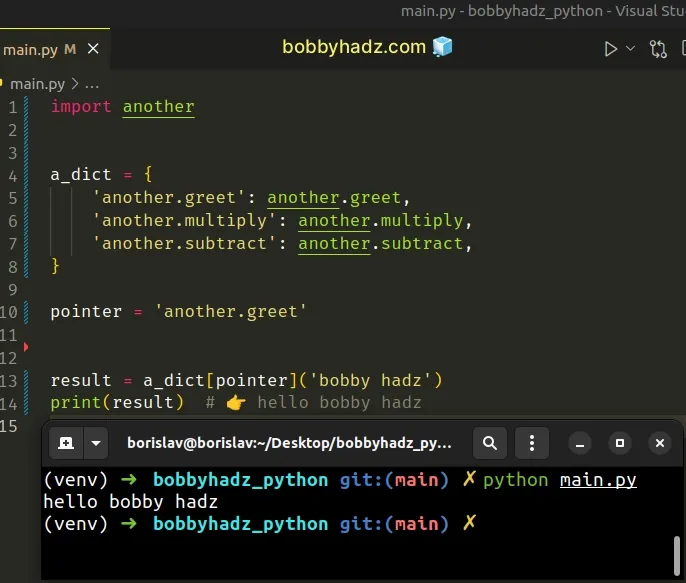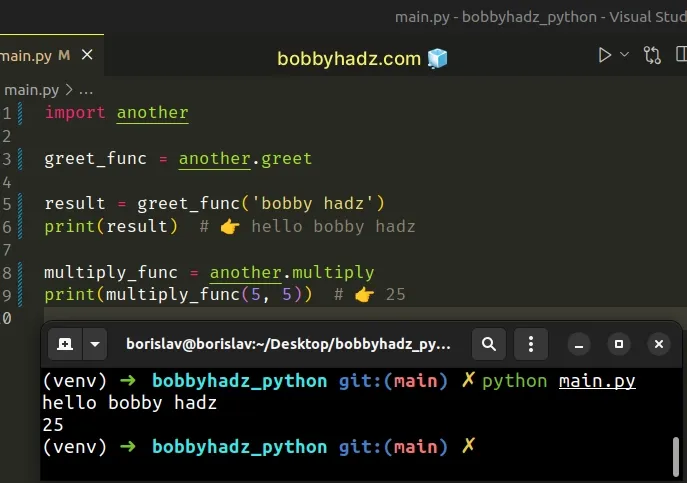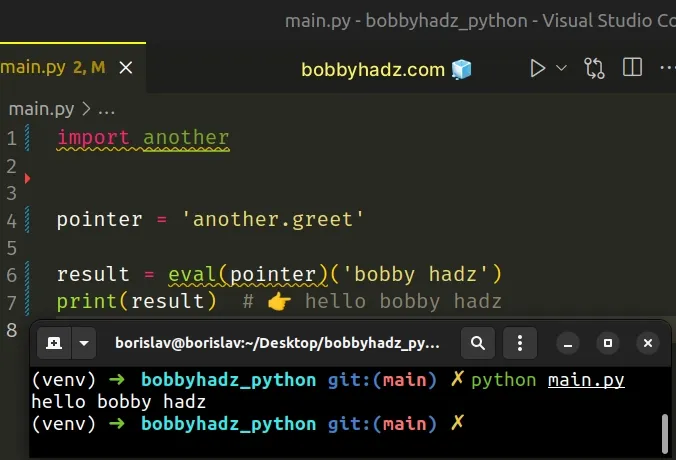# How to use Function Pointers in Python [4 Ways]Last updated: Jul 8, 2023
4 min## #How to use Function Pointers in Python

To use function pointers in Python:

1. Import the module in which the functions are located.
2. Define a dictionary where the keys are the points to the functions and the values are the actual functions.
3. Call the functions by accessing the dictionary keys.

Suppose we have the following `another.py` file.

another.py
```Copied!```def greet(name):
return f'hello {name}'

def multiply(a, b):
return a * b

def subtract(a, b):
return a - b
``````

Here is how you can use function pointers in a file called `main.py`.

main.py
```Copied!```import another

a_dict = {
'another.greet': another.greet,
'another.multiply': another.multiply,
'another.subtract': another.subtract,
}

print(result)  # 👉️ hello bobby hadz

result = a_dict['another.multiply'](5, 5)
print(result)  # 👉️ 25

result = a_dict['another.subtract'](100, 20)
print(result)  # 👉️ 80
``````In some cases, you might also have to start with the name of the package.

main.py
```Copied!```a_dict = {
'package.module.function': package.module.function,
}
``````

We defined 3 functions in the `another.py` module.

The next step is to import the `another` module in `main.py`.

main.py
```Copied!```import another
``````

We then defined a dictionary that contains 3 function pointers - one for each function that is defined in `another.py`.

main.py
```Copied!```a_dict = {
'another.greet': another.greet,
'another.multiply': another.multiply,
'another.subtract': another.subtract,
}
``````

The keys in the dictionary are the pointers and the values are references to the corresponding functions.

Notice that we didn't call the functions with parentheses.

We want to store references to the functions in the dictionary and not the result of calling the functions.

You can then call the functions by accessing the keys in the dictionary.

main.py
```Copied!```result = a_dict['another.greet']('bobby hadz')
print(result)  # 👉️ hello bobby hadz

result = a_dict['another.multiply'](5, 5)
print(result)  # 👉️ 25

result = a_dict['another.subtract'](100, 20)
print(result)  # 👉️ 80
``````

Square brackets `[]` are used to access the specific key and then parentheses are used to invoke the corresponding function.

If the function takes parameters, make sure to supply them between the parentheses.

The same approach can be used if the pointer to the function is stored in a variable.

main.py
```Copied!```import another

a_dict = {
'another.greet': another.greet,
'another.multiply': another.multiply,
'another.subtract': another.subtract,
}

pointer = 'another.greet'

print(result)  # 👉️ hello bobby hadz
``````The `pointer` variable stores the pointer string, so we used it between the square brackets to access the dictionary key.

## #Using function pointers directly in Python

There is also a more direct approach.

The example uses the same `another.py` file.

another.py
```Copied!```def greet(name):
return f'hello {name}'

def multiply(a, b):
return a * b

def subtract(a, b):
return a - b
``````

And here is the related `main.py` file.

main.py
```Copied!```import another

greet_func = another.greet

print(result)  # 👉️ hello bobby hadz
``````In some cases, you might also have to import the module dynamically.

main.py
```Copied!```another_module = __import__('another')

greet_function = getattr(another_module, 'greet')

print(result)  # 👉️ hello bobby hadz
``````

We used the `__import__()` method to import the `another` module and then used the `getattr()` method to get the `greet` function from the module.

I've written a detailed guide on how to call a function by a string name in Python.

We could've also used the importlib.import_module method to achieve the same result.

main.py
```Copied!```import importlib

another_module = importlib.import_module('another')

greet_function = getattr(another_module, 'greet')

print(result)  # 👉️ hello bobby hadz
``````

The method takes a parameter that specifies the module you want to import in absolute or relative terms.

You can also use the str.split() method to split the function pointer string.

main.py
```Copied!```import another
import sys

pointer = 'another.greet'

module_name, func_name = pointer.split('.', 1)

print(result)  # 👉️ hello bobby hadz
``````

The method takes the following 2 parameters:

NameDescription
separatorSplit the string into substrings on each occurrence of the separator
maxsplitAt most `maxsplit` splits are done (optional)

We used a period as the separator and split the pointer string into a list that contains the module name and function name.

The last step is to use the getattr function to access the function based on its name and call it with the second set of parentheses `()`.

## #Using function pointers by calling `eval()` in Python

You can also use the eval() function when using function pointers.

The example uses the same `another.py` file.

another.py
```Copied!```def greet(name):
return f'hello {name}'

def multiply(a, b):
return a * b

def subtract(a, b):
return a - b
``````

And here is the `main.py` file.

main.py
```Copied!```import another

pointer = 'another.greet'

print(result)  # 👉️ hello bobby hadz
``````The function pointer string is stored in a variable.

We called the `eval()` function with the string and it resolved to the actual `greet` function from the `another` module.

The second set of parentheses is used to invoke the `greet` function.

However, notice that we still had to import the `another` module even though it's not directly used in our code.

The argument we passed to the `eval()` function is parsed and evaluated as a Python expression.

The function returns the result of the evaluated expression (the `greet` function in the example).

However, it should be noted that using `eval` with user-supplied data is not safe.

The method simply evaluates the supplied Python string, so passing it an unescaped and insecure user-generated string as a parameter is a cause for security concerns.